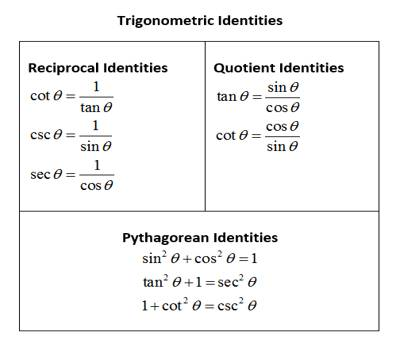A trigonometric identity is a statement involving trigonometric functions which is true for all possible values of the variables involved. Mutual relations among the trigonometrical ratios are generally used to establish the equality of such trigonometrical identities.

When the solutions of any trigonometric ratio problems represent the same expression in the L.H.S. and R.H.S. and the relation is satisfied for all the values of θ then such relation is called a trigonometrical identity.

Verify Trigonometric Identities

To proof and verify the identities we will make use of the basic trigonometric identities to make sure that both the sides of the equation is equal to each other.

1. If tan A = (sin θ – cos θ)/(sin θ + cos θ) then prove that, sin θ + cos θ = ± √2 cos A

Solution:

We know that, sec2 A = 1 + tan2 A

⇒ sec2 A = 1 + (sin θ – cos θ)2/(sin θ + cos θ)2

⇒ sec2 A = [(sin θ + cos θ)2 + (sin θ – cos θ)2]/(sin θ + cos θ)2

⇒ sec2 A = 2(sin2 θ + cos2 θ)/ (sin θ + cos θ)2

⇒ 1/cos2 A = 2/(sin θ + cos θ)2

⇒ (sin θ + cos θ)2 = 2 cos2

Now taking square root on both the sides we get,

sin θ + cos θ =  ± √2 cos A .

ProvedMore examples to get the basic ideas to proof and verify Trigonometric Identities.

1. If x sin3 θ + y cos3 θ = sin θ cos θ and x sin θ – y cos θ = 0, then prove that x2 + y2 = 1, (where, sin θ ≠ 0 and cos θ ≠ 0).

Solution:

x sin θ – y cos θ = 0, (Given)

⇒ x sin θ = y cos θ

⇒ y cos θ = x sin θ

Now dividing both sides by cos θ we get,

y = x ∙ (sin θ/cos θ)

Again, x sin3 θ + y cos3 θ = sin θ cos θ

⇒ x sin3 θ + x ∙ (sin θ /cos θ) ∙ cos3 θ = sin θ cos θ [Since, y = x ∙ (sin θ/cos θ)]

⇒ x sin θ ( sin2 θ + cos2 θ) = sin θ cos θ, [since, cos θ ≠ 0]

⇒ x sin θ (1) = sin θ cos θ,[since, sin2 θ + cos2 θ = 0]

⇒ x sin θ = sin θ cos θ

Now dividing both sides by sin θ we get,

⇒ x = cos θ, [since, sin θ ≠ 0]

Therefore, y = x ∙ (sin θ/cos θ)

⇒ y = cos θ ∙ (sin θ/cos θ), [Putting x = cos θ]

⇒ y = sin θ

Now, x2 + y2

= cos2 θ + sin2 θ = 1.

Therefore, x2 + y2 = 1.

Proved

Information Source: